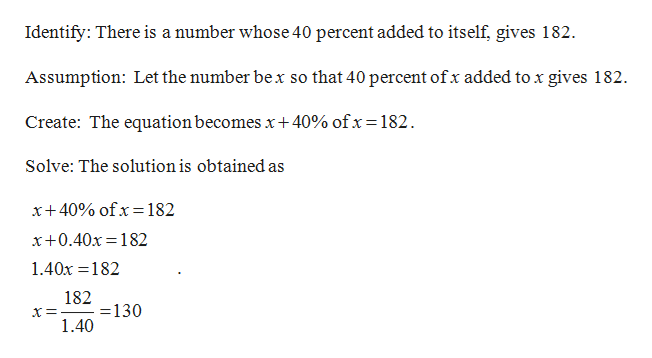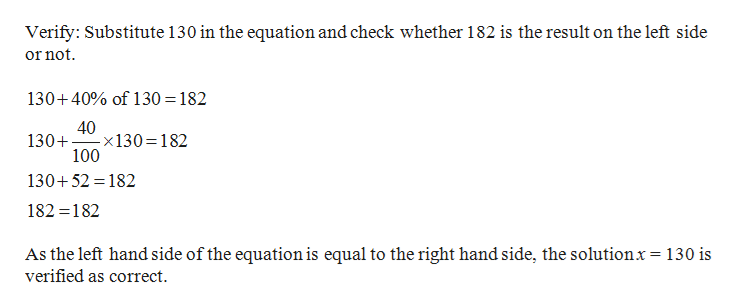Use the​ five-step strategy for solving word problems to find the number or numbers described in the following exercise.When 40​% of a number is added to the​ number, the result is182.What is the​ number?

Question
Use the​ five-step strategy for solving word problems to find the number or numbers described in the following exercise.When 40​% of a number is added to the​ number, the result is182.
What is the​ number?

Step 1help_outlineImage TranscriptioncloseIdentify: There is a number whose 40 percent added to itself, gives 182 Assumption: Let the number bex so that 40 percent of x added to x gives 182 Create: The equation becomes x+40% ofx= 182 Solve: The solution is obtained as x+ 40% ofx= 182 x+0.40x 182 1.40x 182 182 130 1.40 fullscreen
Step 2help_outlineImage TranscriptioncloseVerify: Substitute 130 in the equation and check whether 182 is the result on the left side or not 130+40% of 130 182 40 -x130 182 100 130+ 130+52 182 182 182 As the left hand side of the equation is equal to the right hand side, the solutionx= 130 is verified as correct fullscreen

Want to see the full answer?

See Solution

Want to see this answer and more?

Our solutions are written by experts, many with advanced degrees, and available 24/7

See Solution
Tagged in

Algebra